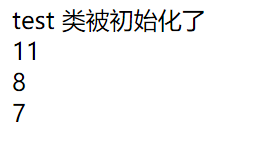# spl_autoload_register 和 __autoload()魔术方法

autoload_function

throw

prepend

test.php

<?php
class test
{
function __construct(){
echo "test 类被初始化了<br>";
}
function show(){
//当前页面是utf8编码
$str = '中文89PHP'; echo strlen($str)."<br/>"; //中文+数字+英文:6 + 2 + 3 = 11
echo mb_strlen($str, 'gbk')."<br/>"; //中文+数字+英文:3 + 2 + 3 = 8 echo mb_strlen($str, 'utf8')."<br/>"; //中文+数字+英文:2 + 2 + 3 = 7

//数据库 varchar 一个中文占一个字符长度
}
}
my_autoload.php 文件
<?php
//方法一:自动加载函数 5.0之后弃用
/*function __autoload($class) {$file = $class . '.php'; if ( is_file($file) ) {
require_once($file); } }$test = new test(); //输出：test 类被初始化了
*/

//使用 spl_autoload_register 函数
//方法二：
class my_autoload
{
public static function autoload($class){$file = $class . '.php'; if ( is_file($file) ) {
require_once($file); } } } //注册加载 spl_autoload_register("my_autoload::autoload",true,true);$test = new test(); //输出：test 类被初始化了
\$test->show();

?>posted @ 2019-05-26 16:36  王默默  阅读(989)  评论(0编辑  收藏  举报